Courses

# Schrodinger Equation(Time-dependent and Time-Independent) - CSIR-NET Physical Science Physics Notes | EduRev

## Physics for IIT JAM, UGC - NET, CSIR NET

Created by: Akhilesh Thakur

## Physics : Schrodinger Equation(Time-dependent and Time-Independent) - CSIR-NET Physical Science Physics Notes | EduRev

The document Schrodinger Equation(Time-dependent and Time-Independent) - CSIR-NET Physical Science Physics Notes | EduRev is a part of the Physics Course Physics for IIT JAM, UGC - NET, CSIR NET.
All you need of Physics at this link: Physics

Time Dependent Schrodinger Equation

The time dependent Schrodinger equation for one spatial dimension is of the form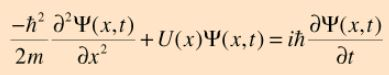For a free particle where U(x) =0 the wavefunction solution can be put in the form of a plane wave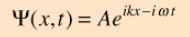For other problems, the potential U(x) serves to set boundary conditions on the spatial part of the wavefunction and it is helpful to separate the equation into the time-independent Schrodinger equation and the relationship for time evolution of the wavefunction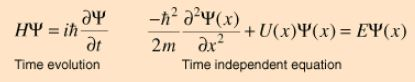Free-Particle Wave Function

For a free particle the time-dependent Schrodinger equation takes the form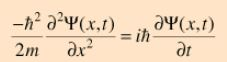and given the dependence upon both position and time, we try a wavefunction of the form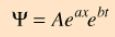Presuming that the wavefunction represents a state of definite energy E, the equation can be separated by the requirement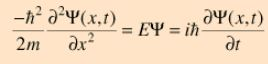Proceeding separately for the position and time equations and taking the indicated derivatives: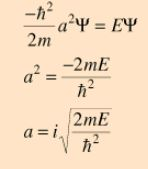Treating the system as a particle where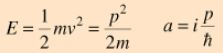Now using the De Broglierelationship and the wave relationship: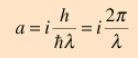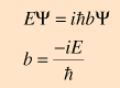Treating the system as a wave packet, or photon-like entity where the Planck hypothesis gives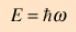we can evaluate the constant b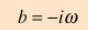This gives a plane wave solution: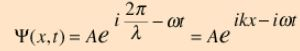Free Particle Waves

The general free-particle wavefunction is of the form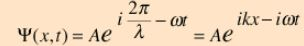which as a complex function can be expanded in the form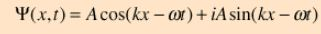Either the real or imaginary part of this function could be appropriate for a given application. In general, one is interested in particles which are free within some kind of boundary, but have boundary conditions set by some kind of potential. The particle in a box problem is the simplest example.

The free particle wavefunction is associated with a precisely known momentum: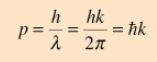but the requirement for normalization makes the wave amplitude approach zero as the wave extends to infinity (uncertainty principle).

Time Independent Schrodinger Equation

The time independent Schrodinger equation for one dimension is of the form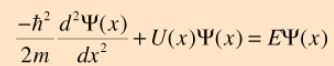where U(x) is the potential energy and E represents the system energy. It is readily generalized to three dimensions, and is often used in spherical polar coordinates.

Energy Eigenvalues

To obtain specific values for energy, you operate on the wavefunction with the quantum mechanical operator associated with energy, which is called the Hamiltonian. The operation of the Hamiltonian on the wavefunction is the Schrodinger equation. Solutions exist for the time-independent Schrodinger equationonly for certain values of energy, and these values are called "eigenvalues" of energy.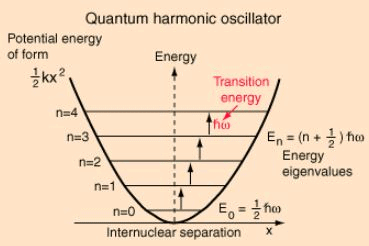For example, the energy eigenvalues of the quantum harmonic oscillator are given by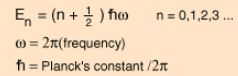The lower vibrational states of diatomic molecules often fit the quantum harmonic oscillator model with sufficient accuracy to permit the determination of bond force constants for the molecules.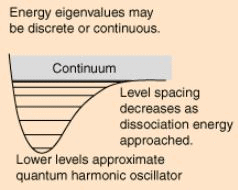While the energy eigenvalues may be discrete for small values of energy, they usually become continuous at high enough energies because the system can no longer exist as a bound state. For a more realistic harmonic oscillator potential (perhaps representing a diatomic molecule), the energy eigenvalues get closer and closer together as it approaches the dissociation energy. The energy levels after dissociation can take the continuous values associated with free particles.

1-D Schrodinger Equation

The time-independent Schrodinger equation is useful for finding energy values for a one dimensional system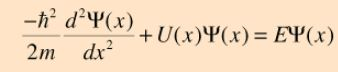Conceptual comments

This equation is useful for the particle in a box problem which yields: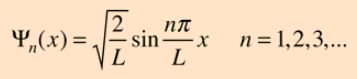To evaluate barrier penetration, the wavefunction inside a barrier is calculated to be of form: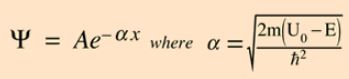The quantum harmonic oscillator in one dimension yields: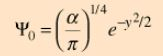This is the ground state wavefunction, where y is the displacement from equilibrium.

159 docs

,

,

,

,

,

,

,

,

,

,

,

,

,

,

,

,

,

,

,

,

,

;